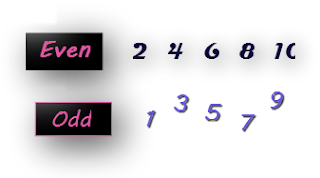Showing posts with label Even Odd Numbers. Show all posts
Showing posts with label Even Odd Numbers. Show all posts## Array with Even and Odd numbers

This is one of the interesting interview question which asked about integer array. Before going into direct question please refer to other tutorial about Java Array which we discussed earlier.

There is an integer array with combination of Even and Odd numbers. Numbers are un-ordered and need to arrange all even numbers left and all odd numbers at right side with ascending order respectively ie., all even numbers at left side and all odd numbers at right should to sorted separately. For example if the input array is {4,6,1,5,8,3,2,7,9}, then output should be {2,4,6,8,1,3,5,7,9}
The constraints are
• Should not use any additional array
• Should not use any collection API
Need to arrange and sort integers in same array. Lets see Java solution for this program by sorting and arranging integers.

```public class EvenOddArray {

public static void main(String[] args) {

int[] array = new int[] {31, 30, 4, 6, 8, 3, 2, 7, 9, 12, 34, 11 };

int evenPnt = 0;

// Arranging Even and Odd numbers
for (int i = 0; i < array.length; i++) {
if (array[i] % 2 == 0) {
swap(array, evenPnt, i);
evenPnt++;
}
}

// Sorting even numbers
sort(array, 0, evenPnt);

// Sorting Odd numbers
sort(array, evenPnt, array.length);

for (int i : array) {
System.out.print(i+", ");
}
}

public static void sort(int[] arr, int min, int max) {
for (int i = min; i < (max - 1); i++) {
for (int j = i + 1; j < max; j++) {
if (arr[i] > arr[j]) {
swap(arr, i, j);
}
}
}
}

public static void swap(int[] arr, int i, int j) {
int tmp = arr[i];
arr[i] = arr[j];
arr[j] = tmp;
}
}
```

OUTPUT:

```2, 4, 6, 8, 12, 30, 34, 3, 7, 9, 11, 31,
```